# Count touching neighbors#

In this notebook we visualize which cells in a tissue are neighbord by drawing a mesh. Furthermore, we can change the intensity of the mesh so that it corresponds to the distance between the centroids of the cells.

import pyclesperanto_prototype as cle
import numpy as np
import pandas as pd


# Test data#

Let’s generate some tissue-like structure consisting of cells which typically have approximately 6 neighbors.

cells = cle.artificial_tissue_2d(
delta_x=48,
delta_y=32,
random_sigma_x=7,
random_sigma_y=7,
width=250,
height=250)

cle.imshow(cells, labels=True)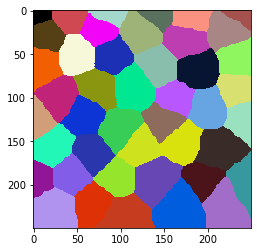## Mesh between neighboring cells#

Before counting neighbors, we should visualize neighbor-relationships. We can do this by drawing a mesh between centroids of touching neighbor cells.

mesh = cle.draw_mesh_between_touching_labels(cells)

cle.imshow(mesh)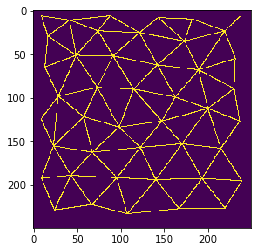We can also combine both visualizations in one image. Note, these images should not be used any further for quantitative analysis. It just serves visualization purposes.

## Centroid connections and cell borders#

A common way for visualizing tissues in this context is by drawing cell-borders and the centroid mesh in different colours.

visualization = mesh * 2 + cle.detect_label_edges(cells)

cle.imshow(visualization, color_map='jet')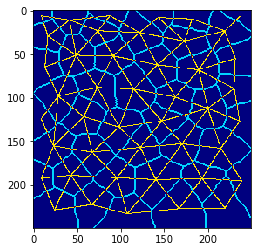## Analyze and visualize number of touching neighbors#

We can also count the touching neighbors and visualize the result as parametric image in colours.

neighbor_count_image = cle.touching_neighbor_count_map(cells)

cle.imshow(neighbor_count_image, color_map='jet', colorbar=True, min_display_intensity=0)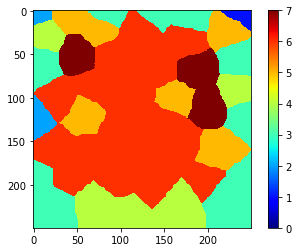Note, the numbers along the image border may not be accurate. Hence, we should exclude the corresponding cells from the further analysis.

cells_ex_border = cle.exclude_labels_on_edges(cells)

cle.imshow(cells_ex_border, labels=True)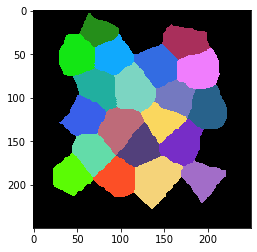After correcting the label image, we can also correct the parametric image.

neighbor_count_image_ex_border = neighbor_count_image * (cells_ex_border != 0)

cle.imshow(neighbor_count_image_ex_border, color_map='jet', colorbar=True, min_display_intensity=0)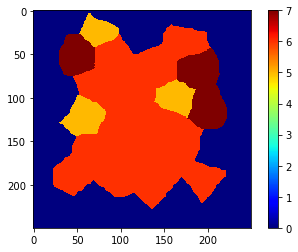Now, we can measure the number of neighbors. We can either just read those numbers and put them in a list …

cle.read_intensities_from_map(cells_ex_border, neighbor_count_image_ex_border)

cl.Array([[0., 5., 6., 7., 6., 6., 7., 6., 6., 5., 5., 6., 6., 7., 6., 6.,
6., 6., 6., 6., 6.]], dtype=float32)


… we can also read these values together with all other statistics and put them in a pandas DataFrame.

statistics = cle.statistics_of_labelled_pixels(neighbor_count_image_ex_border, cells_ex_border)

table = pd.DataFrame(statistics)

# rename a column
table = table.rename(columns={"mean_intensity": "number_of_neighbors"})

# only filter out a subset of all columns; only what we care
table = table[["label", "number_of_neighbors", "centroid_x", "centroid_y"]]

table

label number_of_neighbors centroid_x centroid_y
0 1 5.0 74.592697 23.731028
1 2 6.0 173.741379 35.582230
2 3 7.0 49.460915 51.928082
3 4 6.0 91.783768 52.666199
4 5 6.0 142.728210 62.962471
5 6 7.0 189.973206 68.526794
6 7 6.0 73.699181 88.797356
7 8 6.0 115.321083 90.665649
8 9 5.0 163.097733 99.824242
9 10 5.0 58.206852 122.341309
10 11 6.0 98.847565 134.280228
11 12 6.0 147.731705 127.326218
12 13 7.0 200.522903 112.817368
13 14 6.0 67.880905 162.991592
14 15 6.0 122.943306 157.515717
15 16 6.0 171.010025 153.333328
16 17 6.0 43.426266 189.492981
17 18 6.0 96.272728 192.326477
18 19 6.0 140.815964 194.446030
19 20 6.0 193.817596 193.019318

# Exercise#

Analyze a larger field of view with more cells and vary the parameters random_sigma_x and random_sigma_y of the artificial_tissue_2d function. Use a touching-neighbor-count map to count the number of touching neighbors before and after applying a median filter to the map.